• 今天小编就为大家分享一篇将tensorflow模型打包成PB文件PB文件读取方式，具有很好的参考价值，希望对大家有所帮助。一起跟随小编过来看看吧
• npy文件转为pb文件 下载后 直接打开代码就可以运行 里面包含要转换的npy文件和已经转换好的pb文件 转换完后测试pb文件正确可用
• ## tensorflow实现将ckpt转pb文件

万次阅读 多人点赞 2018-09-01 07:32:30
tensorflow实现将ckpt转pb文件 【尊重原创，转载请注明出处】：https://blog.csdn.net/guyuealian/article/details/82218092 本博客实现将自己训练保存的ckpt模型转换为pb文件，该方法适用于任何ckpt模型，当然你...
tensorflow实现将ckpt转pb文件
【尊重原创，转载请注明出处】：https://blog.csdn.net/guyuealian/article/details/82218092
本博客实现将自己训练保存的ckpt模型转换为pb文件，该方法适用于任何ckpt模型，当然你需要确定ckpt模型输入/输出的节点名称。

目录
tensorflow实现将ckpt转pb文件
一、CKPT 转换成 PB格式
二、 pb模型预测
三、源码下载和资料推荐
1、训练方法
2、本博客Github地址
3、将模型移植Android的方法

使用 tf.train.saver()保存模型时会产生多个文件，会把计算图的结构和图上参数取值分成了不同的文件存储。这种方法是在TensorFlow中是最常用的保存方式。
例如：下面的代码运行后，会在save目录下保存了四个文件：
import tensorflow as tf
# 声明两个变量
v1 = tf.Variable(tf.random_normal([1, 2]), name="v1")
v2 = tf.Variable(tf.random_normal([2, 3]), name="v2")
init_op = tf.global_variables_initializer() # 初始化全部变量
saver = tf.train.Saver() # 声明tf.train.Saver类用于保存模型
with tf.Session() as sess:
sess.run(init_op)
print("v1:", sess.run(v1)) # 打印v1、v2的值一会读取之后对比
print("v2:", sess.run(v2))
saver_path = saver.save(sess, "save/model.ckpt")  # 将模型保存到save/model.ckpt文件
print("Model saved in file:", saver_path)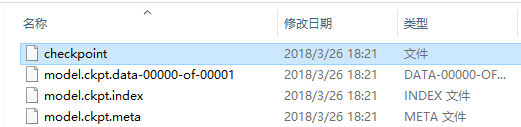其中

checkpoint是检查点文件，文件保存了一个目录下所有的模型文件列表；model.ckpt.meta文件保存了TensorFlow计算图的结构，可以理解为神经网络的网络结构，该文件可以被 tf.train.import_meta_graph 加载到当前默认的图来使用。ckpt.data ： 保存模型中每个变量的取值

但很多时候，我们需要将TensorFlow的模型导出为单个文件（同时包含模型结构的定义与权重），方便在其他地方使用（如在Android中部署网络）。利用tf.train.write_graph()默认情况下只导出了网络的定义（没有权重），而利用tf.train.Saver().save()导出的文件graph_def与权重是分离的，因此需要采用别的方法。 我们知道，graph_def文件中没有包含网络中的Variable值（通常情况存储了权重），但是却包含了constant值，所以如果我们能把Variable转换为constant，即可达到使用一个文件同时存储网络架构与权重的目标。
TensoFlow为我们提供了convert_variables_to_constants()方法，该方法可以固化模型结构，将计算图中的变量取值以常量的形式保存，而且保存的模型可以移植到Android平台。

一、CKPT 转换成 PB格式
将CKPT 转换成 PB格式的文件的过程可简述如下：

通过传入 CKPT 模型的路径得到模型的图和变量数据通过 import_meta_graph 导入模型中的图通过 saver.restore 从模型中恢复图中各个变量的数据通过 graph_util.convert_variables_to_constants 将模型持久化

def freeze_graph(input_checkpoint,output_graph):
'''

:param input_checkpoint:
:param output_graph: PB模型保存路径
:return:
'''
# checkpoint = tf.train.get_checkpoint_state(model_folder) #检查目录下ckpt文件状态是否可用
# input_checkpoint = checkpoint.model_checkpoint_path #得ckpt文件路径

# 指定输出的节点名称,该节点名称必须是原模型中存在的节点
output_node_names = "InceptionV3/Logits/SpatialSqueeze"
saver = tf.train.import_meta_graph(input_checkpoint + '.meta', clear_devices=True)
graph = tf.get_default_graph() # 获得默认的图
input_graph_def = graph.as_graph_def()  # 返回一个序列化的图代表当前的图

with tf.Session() as sess:
saver.restore(sess, input_checkpoint) #恢复图并得到数据
output_graph_def = graph_util.convert_variables_to_constants(  # 模型持久化，将变量值固定
sess=sess,
input_graph_def=input_graph_def,# 等于:sess.graph_def
output_node_names=output_node_names.split(","))# 如果有多个输出节点，以逗号隔开

with tf.gfile.GFile(output_graph, "wb") as f: #保存模型
f.write(output_graph_def.SerializeToString()) #序列化输出
print("%d ops in the final graph." % len(output_graph_def.node)) #得到当前图有几个操作节点

# for op in graph.get_operations():
#     print(op.name, op.values())
说明：

1、函数freeze_graph中，最重要的就是要确定“指定输出的节点名称”，这个节点名称必须是原模型中存在的节点，对于freeze操作，我们需要定义输出结点的名字。因为网络其实是比较复杂的，定义了输出结点的名字，那么freeze的时候就只把输出该结点所需要的子图都固化下来，其他无关的就舍弃掉。因为我们freeze模型的目的是接下来做预测。所以，output_node_names一般是网络模型最后一层输出的节点名称，或者说就是我们预测的目标。
2、在保存的时候，通过convert_variables_to_constants函数来指定需要固化的节点名称，对于鄙人的代码，需要固化的节点只有一个：output_node_names。注意节点名称与张量的名称的区别，例如：“input:0”是张量的名称，而"input"表示的是节点的名称。
3、源码中通过graph = tf.get_default_graph()获得默认的图，这个图就是由saver = tf.train.import_meta_graph(input_checkpoint + '.meta', clear_devices=True)恢复的图，因此必须先执行tf.train.import_meta_graph，再执行tf.get_default_graph() 。
4、实质上，我们可以直接在恢复的会话sess中，获得默认的网络图，更简单的方法，如下：

def freeze_graph(input_checkpoint,output_graph):
'''

:param input_checkpoint:
:param output_graph: PB模型保存路径
:return:
'''
# checkpoint = tf.train.get_checkpoint_state(model_folder) #检查目录下ckpt文件状态是否可用
# input_checkpoint = checkpoint.model_checkpoint_path #得ckpt文件路径

# 指定输出的节点名称,该节点名称必须是原模型中存在的节点
output_node_names = "InceptionV3/Logits/SpatialSqueeze"
saver = tf.train.import_meta_graph(input_checkpoint + '.meta', clear_devices=True)

with tf.Session() as sess:
saver.restore(sess, input_checkpoint) #恢复图并得到数据
output_graph_def = graph_util.convert_variables_to_constants(  # 模型持久化，将变量值固定
sess=sess,
input_graph_def=sess.graph_def,# 等于:sess.graph_def
output_node_names=output_node_names.split(","))# 如果有多个输出节点，以逗号隔开

with tf.gfile.GFile(output_graph, "wb") as f: #保存模型
f.write(output_graph_def.SerializeToString()) #序列化输出
print("%d ops in the final graph." % len(output_graph_def.node)) #得到当前图有几个操作节点
调用方法很简单，输入ckpt模型路径，输出pb模型的路径即可：
    # 输入ckpt模型路径
input_checkpoint='models/model.ckpt-10000'
# 输出pb模型的路径
out_pb_path="models/pb/frozen_model.pb"
# 调用freeze_graph将ckpt转为pb
freeze_graph(input_checkpoint,out_pb_path)

5、上面以及说明：在保存的时候，通过convert_variables_to_constants函数来指定需要固化的节点名称，对于鄙人的代码，需要固化的节点只有一个：output_node_names。因此，其他网络模型，也可以通过简单的修改输出的节点名称output_node_names，将ckpt转为pb文件 。
PS：注意节点名称，应包含name_scope 和 variable_scope命名空间，并用“/”隔开，如"InceptionV3/Logits/SpatialSqueeze"

二、 pb模型预测
下面是预测pb模型的代码

def freeze_graph_test(pb_path, image_path):
'''
:param pb_path:pb文件的路径
:param image_path:测试图片的路径
:return:
'''
with tf.Graph().as_default():
output_graph_def = tf.GraphDef()
with open(pb_path, "rb") as f:
tf.import_graph_def(output_graph_def, name="")
with tf.Session() as sess:
sess.run(tf.global_variables_initializer())

# 定义输入的张量名称,对应网络结构的输入张量
# input:0作为输入图像,keep_prob:0作为dropout的参数,测试时值为1,is_training:0训练参数
input_image_tensor = sess.graph.get_tensor_by_name("input:0")
input_keep_prob_tensor = sess.graph.get_tensor_by_name("keep_prob:0")
input_is_training_tensor = sess.graph.get_tensor_by_name("is_training:0")

# 定义输出的张量名称
output_tensor_name = sess.graph.get_tensor_by_name("InceptionV3/Logits/SpatialSqueeze:0")

# 读取测试图片
im=im[np.newaxis,:]
# 测试读出来的模型是否正确，注意这里传入的是输出和输入节点的tensor的名字，不是操作节点的名字
# out=sess.run("InceptionV3/Logits/SpatialSqueeze:0", feed_dict={'input:0': im,'keep_prob:0':1.0,'is_training:0':False})
out=sess.run(output_tensor_name, feed_dict={input_image_tensor: im,
input_keep_prob_tensor:1.0,
input_is_training_tensor:False})
print("out:{}".format(out))
score = tf.nn.softmax(out, name='pre')
class_id = tf.argmax(score, 1)
print "pre class_id:{}".format(sess.run(class_id))
说明：

1、与ckpt预测不同的是，pb文件已经固化了网络模型结构，因此，即使不知道原训练模型（train）的源码，我们也可以恢复网络图，并进行预测。恢复模型十分简单，只需要从读取的序列化数据中导入网络结构即可：
tf.import_graph_def(output_graph_def, name="")

2、但必须知道原网络模型的输入和输出的节点名称（当然了，传递数据时，是通过输入输出的张量来完成的）。由于InceptionV3模型的输入有三个节点，因此这里需要定义输入的张量名称，它对应网络结构的输入张量：
input_image_tensor = sess.graph.get_tensor_by_name("input:0")
input_keep_prob_tensor = sess.graph.get_tensor_by_name("keep_prob:0")
input_is_training_tensor = sess.graph.get_tensor_by_name("is_training:0")
以及输出的张量名称：
output_tensor_name = sess.graph.get_tensor_by_name("InceptionV3/Logits/SpatialSqueeze:0")

3、预测时，需要feed输入数据：
# 测试读出来的模型是否正确，注意这里传入的是输出和输入节点的tensor的名字，不是操作节点的名字
# out=sess.run("InceptionV3/Logits/SpatialSqueeze:0", feed_dict={'input:0': im,'keep_prob:0':1.0,'is_training:0':False})
out=sess.run(output_tensor_name, feed_dict={input_image_tensor: im,
input_keep_prob_tensor:1.0,
input_is_training_tensor:False})
4、其他网络模型预测时，也可以通过修改输入和输出的张量的名称 。
PS：注意张量的名称，即为：节点名称+“:”+“id号”，如"InceptionV3/Logits/SpatialSqueeze:0"

完整的CKPT 转换成 PB格式和预测的代码如下：
# -*-coding: utf-8 -*-
"""
@Project: tensorflow_models_nets
@File   : convert_pb.py
@Author : panjq
@E-mail : pan_jinquan@163.com
@Date   : 2018-08-29 17:46:50
@info   :
-通过传入 CKPT 模型的路径得到模型的图和变量数据
-通过 import_meta_graph 导入模型中的图
-通过 saver.restore 从模型中恢复图中各个变量的数据
-通过 graph_util.convert_variables_to_constants 将模型持久化
"""

import tensorflow as tf
from create_tf_record import *
from tensorflow.python.framework import graph_util

resize_height = 299  # 指定图片高度
resize_width = 299  # 指定图片宽度
depths = 3

def freeze_graph_test(pb_path, image_path):
'''
:param pb_path:pb文件的路径
:param image_path:测试图片的路径
:return:
'''
with tf.Graph().as_default():
output_graph_def = tf.GraphDef()
with open(pb_path, "rb") as f:
tf.import_graph_def(output_graph_def, name="")
with tf.Session() as sess:
sess.run(tf.global_variables_initializer())

# 定义输入的张量名称,对应网络结构的输入张量
# input:0作为输入图像,keep_prob:0作为dropout的参数,测试时值为1,is_training:0训练参数
input_image_tensor = sess.graph.get_tensor_by_name("input:0")
input_keep_prob_tensor = sess.graph.get_tensor_by_name("keep_prob:0")
input_is_training_tensor = sess.graph.get_tensor_by_name("is_training:0")

# 定义输出的张量名称
output_tensor_name = sess.graph.get_tensor_by_name("InceptionV3/Logits/SpatialSqueeze:0")

# 读取测试图片
im=im[np.newaxis,:]
# 测试读出来的模型是否正确，注意这里传入的是输出和输入节点的tensor的名字，不是操作节点的名字
# out=sess.run("InceptionV3/Logits/SpatialSqueeze:0", feed_dict={'input:0': im,'keep_prob:0':1.0,'is_training:0':False})
out=sess.run(output_tensor_name, feed_dict={input_image_tensor: im,
input_keep_prob_tensor:1.0,
input_is_training_tensor:False})
print("out:{}".format(out))
score = tf.nn.softmax(out, name='pre')
class_id = tf.argmax(score, 1)
print "pre class_id:{}".format(sess.run(class_id))

def freeze_graph(input_checkpoint,output_graph):
'''

:param input_checkpoint:
:param output_graph: PB模型保存路径
:return:
'''
# checkpoint = tf.train.get_checkpoint_state(model_folder) #检查目录下ckpt文件状态是否可用
# input_checkpoint = checkpoint.model_checkpoint_path #得ckpt文件路径

# 指定输出的节点名称,该节点名称必须是原模型中存在的节点
output_node_names = "InceptionV3/Logits/SpatialSqueeze"
saver = tf.train.import_meta_graph(input_checkpoint + '.meta', clear_devices=True)

with tf.Session() as sess:
saver.restore(sess, input_checkpoint) #恢复图并得到数据
output_graph_def = graph_util.convert_variables_to_constants(  # 模型持久化，将变量值固定
sess=sess,
input_graph_def=sess.graph_def,# 等于:sess.graph_def
output_node_names=output_node_names.split(","))# 如果有多个输出节点，以逗号隔开

with tf.gfile.GFile(output_graph, "wb") as f: #保存模型
f.write(output_graph_def.SerializeToString()) #序列化输出
print("%d ops in the final graph." % len(output_graph_def.node)) #得到当前图有几个操作节点

# for op in sess.graph.get_operations():
#     print(op.name, op.values())

def freeze_graph2(input_checkpoint,output_graph):
'''

:param input_checkpoint:
:param output_graph: PB模型保存路径
:return:
'''
# checkpoint = tf.train.get_checkpoint_state(model_folder) #检查目录下ckpt文件状态是否可用
# input_checkpoint = checkpoint.model_checkpoint_path #得ckpt文件路径

# 指定输出的节点名称,该节点名称必须是原模型中存在的节点
output_node_names = "InceptionV3/Logits/SpatialSqueeze"
saver = tf.train.import_meta_graph(input_checkpoint + '.meta', clear_devices=True)
graph = tf.get_default_graph() # 获得默认的图
input_graph_def = graph.as_graph_def()  # 返回一个序列化的图代表当前的图

with tf.Session() as sess:
saver.restore(sess, input_checkpoint) #恢复图并得到数据
output_graph_def = graph_util.convert_variables_to_constants(  # 模型持久化，将变量值固定
sess=sess,
input_graph_def=input_graph_def,# 等于:sess.graph_def
output_node_names=output_node_names.split(","))# 如果有多个输出节点，以逗号隔开

with tf.gfile.GFile(output_graph, "wb") as f: #保存模型
f.write(output_graph_def.SerializeToString()) #序列化输出
print("%d ops in the final graph." % len(output_graph_def.node)) #得到当前图有几个操作节点

# for op in graph.get_operations():
#     print(op.name, op.values())

if __name__ == '__main__':
# 输入ckpt模型路径
input_checkpoint='models/model.ckpt-10000'
# 输出pb模型的路径
out_pb_path="models/pb/frozen_model.pb"
# 调用freeze_graph将ckpt转为pb
freeze_graph(input_checkpoint,out_pb_path)

# 测试pb模型
image_path = 'test_image/animal.jpg'
freeze_graph_test(pb_path=out_pb_path, image_path=image_path)


三、源码下载和资料推荐
1、训练方法

2、本博客Github地址

Github源码：https://github.com/PanJinquan/tensorflow_models_learning中的convert_pb.py文件

3、将模型移植Android的方法
pb文件是可以移植到Android平台运行的，其方法，可参考：

《将tensorflow训练好的模型移植到Android (MNIST手写数字识别)》
https://blog.csdn.net/guyuealian/article/details/79672257


展开全文ckpt转pb
• 今天小编就为大家分享一篇TensorFlow实现checkpoint文件转换为pb文件，具有很好的参考价值，希望对大家有所帮助。一起跟随小编过来看看吧
• 由于要同时运行几个网络,所以打算将这个网络模型进行固化成pb文件,然后直接调用. 主要包括一下内容: 1.查看ckpt模型的输入输出张量名称. 2.将ckpt文件生成pb文件. 3.查看生成的pb文件的输入输出节点 4.运行pb...
前段时间搭建了一个分类网络模型,然后用自己的数据进行了800epoch’的训练,最后默认生成了三个ckpt文件.由于要同时运行几个网络,所以打算将这个网络模型进行固化成pb文件,然后直接调用. 主要包括一下内容:

1.查看ckpt模型的输入输出张量名称.
2.将ckpt文件生成pb文件.
3.查看生成的pb文件的输入输出节点
4.运行pb文件,进行网络预测

1.查看ckpt网络的输入输出张量名称 下面是我的网络训练后生成的三个ckpt文件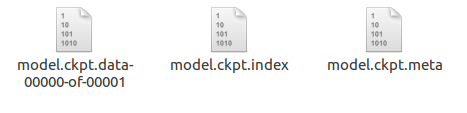运行以下代码查看自己模型的输入输出张量名称(用于保存pb文件时保留这两个节点) 注意第三行代码换成自己的ckpt文件地址,名称是三个文件共有的 model.ckpt
from tensorflow.python import pywrap_tensorflow
import os
checkpoint_path=os.path.join('/media/wsb/King/TEAM/Semantic-Segmentation-Suite/checkpoints/0295/model.ckpt')
for key in var_to_shape_map:
print( 'tensor_name: ',key)
print("a.shape:%s"%[a.shape])

我的代码运行后结果如下: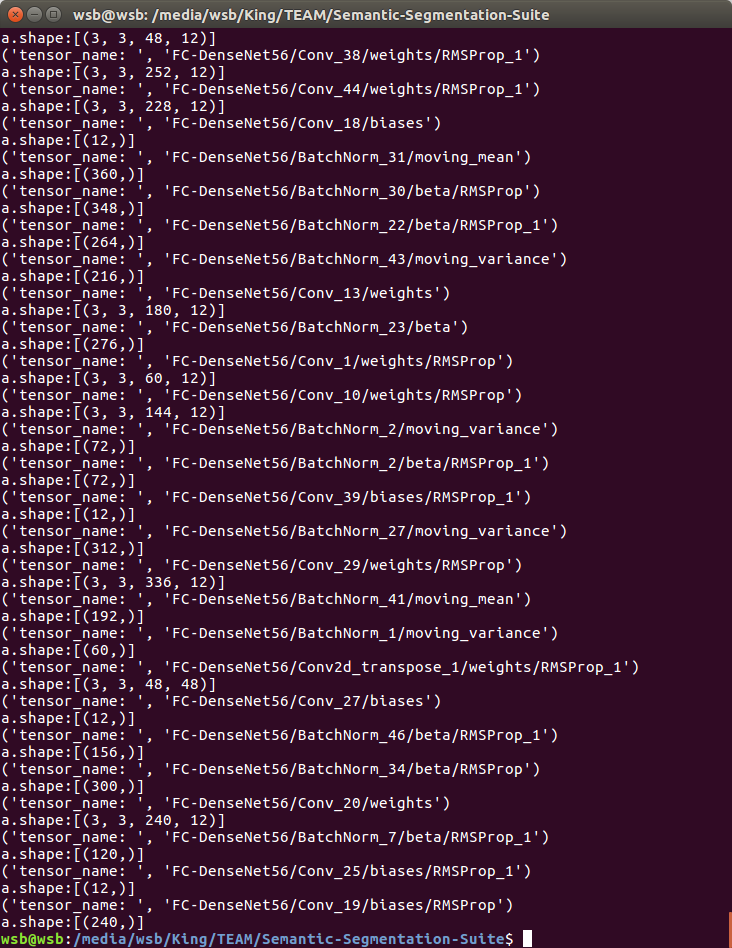如果你的模型输入输出张量很容易找到,那这个方法对于你来说应该是可以的,但是我就是在这里花了一天的时间才找到自己模型的输入输出张量,因为这个模型比较复杂,并且这个程序输出的张量是无序的.我使用的模型是别人语义分割模型的改进,所以模型张量不是很好找. 仍然找不到输入输出张量怎么办? 我的解决办法:我通过程序找到了模型的定义,然后在模型的最前端打印出输入张量,在最后打印出输出张量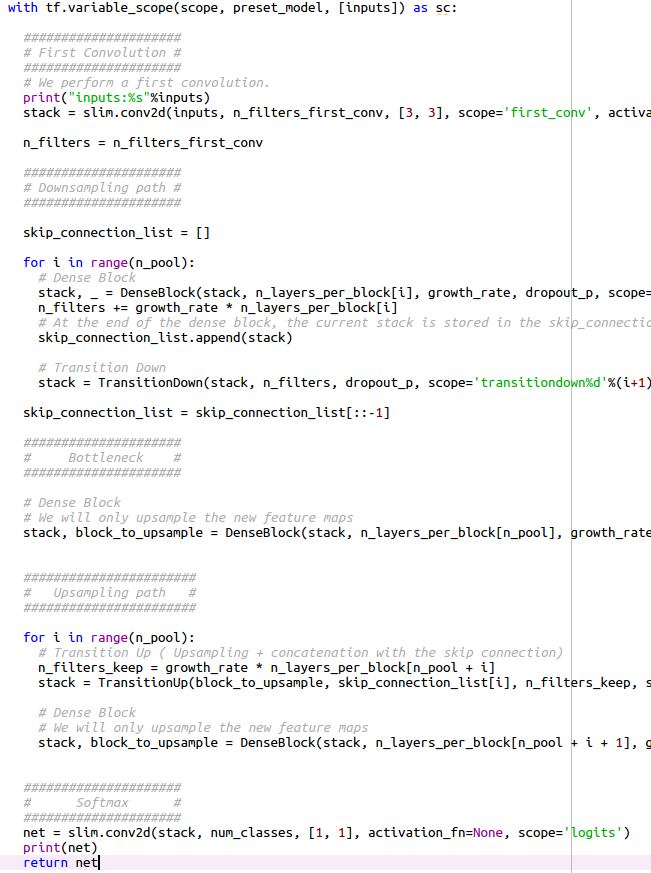上图中的第二行代码是输出"inputs"张量,倒数第二行代码输出"net"张量,然后运行包含模型代码的程序就可以看到打印出来的两个张量了.下图就是运行后的输出结果,这样就找到自己模型的输入和输出张量了.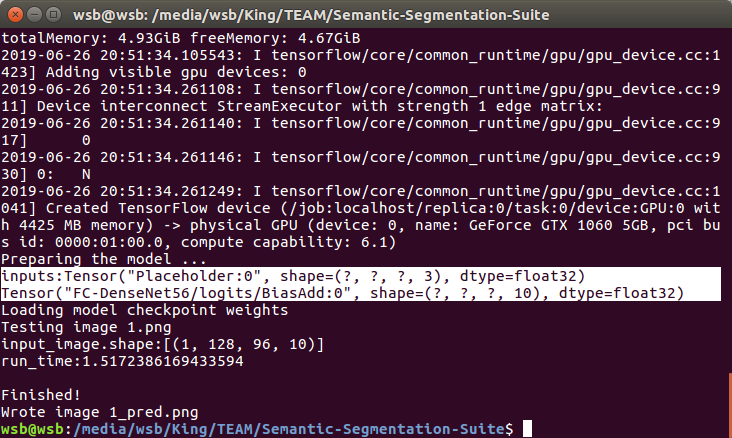2.将ckpt文件生成pb文件. 以下是将ckpt文件转化为pb文件的代码 1)更改node_names后面的值,改成自己想要保留的节点名称,我保留了首尾两个,就是上面得到的两个. 2)input_checkpoint地址改成自己的ckpt文件的地址.(注意写到.ckpt)
import tensorflow as tf
from tensorflow.python.framework import graph_util
def freeze_graph(input_checkpoint,output_graph):
'''
:param input_checkpoint:
:param output_graph: PB模型保存路径
:return:
'''
# 指定输出的节点名称,该节点名称必须是原模型中存在的节点
# 直接用最后输出的节点，可以在tensorboard中查找到，tensorboard只能在linux中使用
saver = tf.train.import_meta_graph(input_checkpoint+".meta" , clear_devices=True)
graph = tf.get_default_graph() # 获得默认的图
input_graph_def = graph.as_graph_def()  # 返回一个序列化的图代表当前的图
init = tf.global_variables_initializer()
with tf.Session() as sess:
sess.run(init)
saver.restore(sess, input_checkpoint) #恢复图并得到数据
output_graph_def = graph_util.convert_variables_to_constants(  # 模型持久化，将变量值固定
sess=sess,
input_graph_def=input_graph_def,# 等于:sess.graph_def
output_node_names=output_node_names.split(","))# 如果有多个输出节点，以逗号隔开

with tf.gfile.GFile(output_graph, "wb") as f: #保存模型
f.write(output_graph_def.SerializeToString()) #序列化输出
print("%d ops in the final graph." % len(output_graph_def.node)) #得到当前图有几个操作节点
input_checkpoint="/media/wsb/King/TEAM/Semantic-Segmentation-Suite/checkpoints/0295/model.ckpt"#输入的ckpt文件位置
output_graph="node.pb"#输出节点的文件名
freeze_graph(input_checkpoint,output_graph)

然后就可以得到一个node.pb文件,名字可以自己更改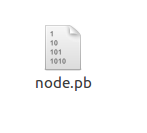3.查看生成的pb文件的输入输出节点 查看pb文件的节点,只是为了验证一下,也可以不查看,代码如下:
只需更改你的pb文件的地址,运行后会得到一个txt文件,打开可以查看
import tensorflow as tf
import os

model_dir = './'
model_name = 'new_node.pb'

def create_graph():
with tf.gfile.FastGFile(os.path.join(
model_dir, model_name), 'rb') as f:
# 使用tf.GraphDef()定义一个空的Graph
graph_def = tf.GraphDef()
# Imports the graph from graph_def into the current default Graph.
tf.import_graph_def(graph_def, name='')

# 创建graph
create_graph()

tensor_name_list = [tensor.name for tensor in tf.get_default_graph().as_graph_def().node]
result_file = os.path.join(model_dir, 'result.txt')
with open(result_file, 'w+') as f:
for tensor_name in tensor_name_list:
f.write(tensor_name+'\n')

下面是我的txt文件的内容,好像我的pb文件生成了整个网络的节点,并不只是保留了输入和输出两个,看一下输入输出节点和刚才查看的是对应的.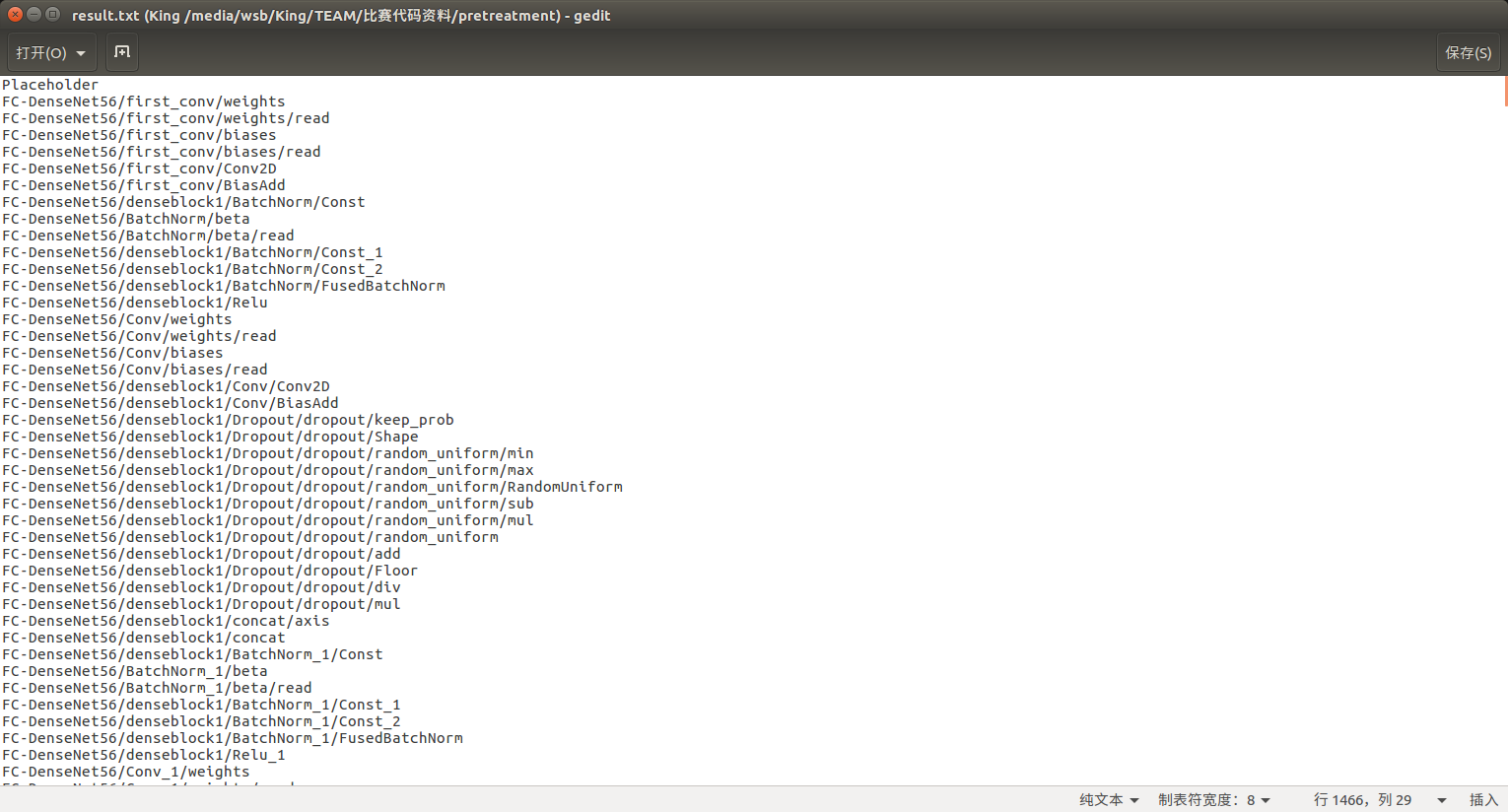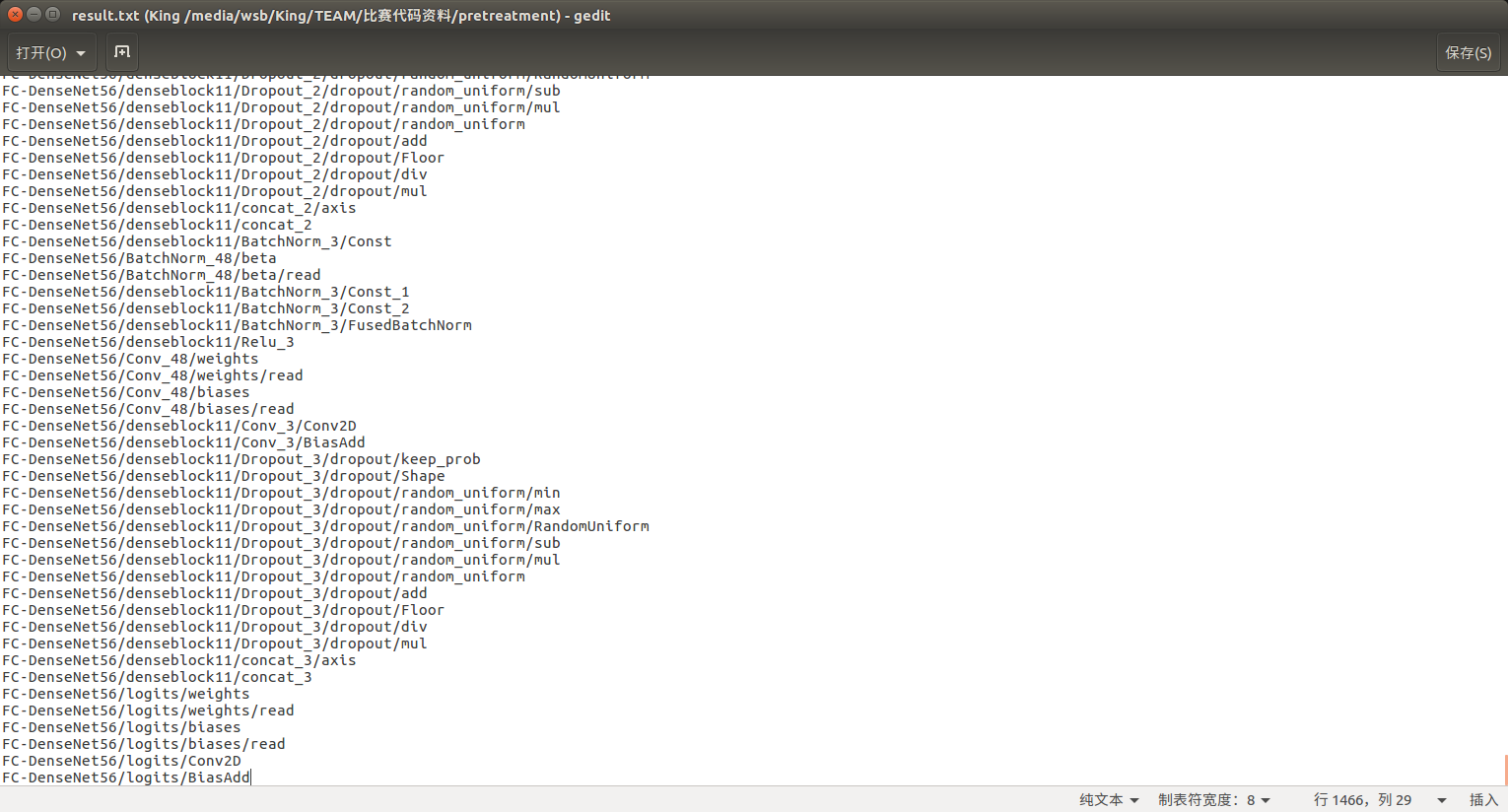4.运行pb文件,进行网络预测 以下是我用自己的pb文件进行我自己图片的预测,代码如下:
def get_RAC(image_path, pb_file_path):
with tf.Graph().as_default():
output_graph_def = tf.GraphDef()

with open(pb_file_path, "rb") as f:
_ = tf.import_graph_def(output_graph_def, name="")

with tf.Session() as sess:
init = tf.global_variables_initializer()
sess.run(init)

input_x = sess.graph.get_tensor_by_name("Placeholder:0")
output_image = sess.run(final_result, feed_dict={input_x: input_x })
return output_image

运行上面代码就可以得到网络的输出结果.
展开全文ckpt
• 1.pb文件pb是protocol（协议） buffer（缓冲）的缩写。TensorFlow训练模型后存成的pb文件，是一种表示模型（神经网络）结构的二进制文件，不带有源代码，也一般无法映射成源代码。这就有点像C 语言编译产生的机器码...
1.pb文件

pb是protocol（协议） buffer（缓冲）的缩写。TensorFlow训练模型后存成的pb文件，是一种表示模型（神经网络）结构的二进制文件，不带有源代码，也一般无法映射成源代码。这就有点像C 语言编译产生的机器码一般无法映射回源代码一样。
pb文件作为SavedModel的一部分，可以加载回TensorFlow进行部署或进一步训练。
作者：蔡善清
来源：知乎
著作权归作者所有。商业转载请联系作者获得授权，非商业转载请注明出处。

以上是在我困惑时，知乎作者的回答，我一直想打开这个pb文件看源代码，后来才知道这里根本没有源代码，要找代码还是去github的作者下找代码，不要再试图打开pb文件了。
2.tensorflow模型的保存与恢复是怎样的呢？
这一部分包括变量的保存和模型的保存
参考官网：tensorflow
tf.train.saver 程序类提供了保存和恢复模型的方法。tf.saved_model.simple_save 函数是构建适合服务的保存模型的简单方法。估计器在model_dir中自动保存和恢复变量。
------------------------------------------------------------------------------------------------------
2.1 保存和恢复变量
保存：用tf.train.Saver()创建一个Saver来管理模型中的所有变量。例如，下面的代码片段演示了如何调用tf.train.Saver。保存方法，将变量保存到检查点文件（checkpoint file).
import tensorflow as tf

# Create some variables.
v1 = tf.get_variable("v1", shape=, initializer = tf.zeros_initializer)
v2 = tf.get_variable("v2", shape=, initializer = tf.zeros_initializer)

inc_v1 = v1.assign(v1+1)
dec_v2 = v2.assign(v2-1)

# Add an op to initialize the variables.
init_op = tf.global_variables_initializer()

# Add ops to save and restore all the variables.
saver = tf.train.Saver()

# Later, launch the model, initialize the variables, do some work, and save the
# variables to disk.
with tf.Session() as sess:
sess.run(init_op)
# Do some work with the model.
inc_v1.op.run()
dec_v2.op.run()
# Save the variables to disk.
save_path = saver.save(sess, "model.ckpt")
print("Model saved in path {}".format(save_path))
恢复：注意，当您恢复变量时，您不需要预先初始化它们。例如，下面的代码片段演示了如何调用tf.train.Saver。恢复从检查点文件中恢复变量的方法:
tf.reset_default_graph()
# 将以前的graph清零

# Create some variables.
v1 = tf.get_variable("v1", shape=)
v2 = tf.get_variable("v2", shape=)

# Add ops to save and restore all the variables.
saver = tf.train.Saver()

# Later, launch the model, use the saver to restore variables from disk, and
# do some work with the model.
with tf.Session() as sess:
# Restore variables from disk.
saver.restore(sess, "model.ckpt")
print("Model restored.")
# Check the values of the variables
print("v1 : %s" % v1.eval())
print("v2 : %s" % v2.eval())
注意：没有一个物理文件叫做model.ckpt。它是为检查点创建的文件名的前缀。用户只与前缀交互，而不与物理检查点文件交互。换句话说，你保存的文件没有model.ckpt这个文件，而是一个叫checkpoint的文件

选择存储和恢复哪些变量
如果你不给tf.train.Saver()传入任何参数，那么saver将处理graph中的所有变量。其中每一个变量都以变量创建时传入的名称被保存。有时候在检查点文件中明确定义变量的名称很有用。举个例子，你也许已经训练得到了一个模型，其中有个变量命名为"weights"，你想把它的值恢复到一个新的变量"params"中。
有时候仅保存和恢复模型的一部分变量很有用。再举个例子，你也许训练得到了一个5层神经网络，现在想训练一个6层的新模型，可以将之前5层模型的参数导入到新模型的前5层中。 你可以通过给tf.train.Saver()构造函数传入Python字典，很容易地定义需要保持的变量及对应名称：键对应使用的名称，值对应被管理的变量。 注意： 1.如果需要保存和恢复模型变量的不同子集，可以创建任意多个saver对象。同一个变量可被列入多个saver对象中，只有当saver的restore()函数被运行时，它的值才会发生改变。
2.如果你仅在session开始时恢复模型变量的一个子集，你需要对剩下的变量执行初始化op。详情请见tf.initialize_variables()。3.要检查检查点中的变量，您可以使用inspect_checkpoint库，特别是print_tensors_in_checkpoint_file 函数
tf.reset_default_graph()
# Create some variables.
v1 = tf.get_variable("v1", , initializer = tf.zeros_initializer)
v2 = tf.get_variable("v2", , initializer = tf.zeros_initializer)

# Add ops to save and restore only v2 using the name "v2"
saver = tf.train.Saver({"v2": v2}) # 单独保存一个变量创建字典，键为名，值为值

# Use the saver object normally after that.
with tf.Session() as sess:
# Initialize v1 since the saver will not.
v1.initializer.run() # 保存的变量 v2 不用初始化，就像是做迁移学习直接用保存的值作为初始值，所以不要再初始化
saver.restore(sess, "model.ckpt")

print("v1 : %s" % v1.eval())
print("v2 : %s" % v2.eval())
下面演示使用print_tensors_in_checkpoint_file（）检查在checkpoint中的变量
# import the inspect_checkpoint library
from tensorflow.python.tools import inspect_checkpoint as chkp

# print all tensors in checkpoint file
chkp.print_tensors_in_checkpoint_file("model.ckpt", tensor_name='', all_tensors=True)

# tensor_name:  v1
# [ 1.  1.  1.]
# tensor_name:  v2
# [-1. -1. -1. -1. -1.]

# print only tensor v1 in checkpoint file
chkp.print_tensors_in_checkpoint_file("model.ckpt", tensor_name='v1', all_tensors=False)

# tensor_name:  v1
# [ 1.  1.  1.]

# print only tensor v2 in checkpoint file
chkp.print_tensors_in_checkpoint_file("model.ckpt", tensor_name='v2', all_tensors=False)

# tensor_name:  v2
# [-1. -1. -1. -1. -1.]
2.2 保存和恢复模型（models）
使用SavedModel来保存和加载您的模型变量、图和图的元数据。这是一种与语言无关的、可恢复的、可恢复的序列化格式，可以支持更高级别的系统和工具来生成、使用和转换TensorFlow模型。TensorFlow提供了几种与SavedModel交互的方法，包括tf.saved_model api、tf.estimator.Estimator和命令行界面。
构建并加载SavedModel：
2.2.1 简单保存
创建SavedModel的最简单方法是使用tf.saved_model.simple_save 函数
simple_save(session,
export_dir,
inputs={"x": x, "y": y},
outputs={"z": z})
2.2.2 手工构建一个SavedModel
使用 tf.saved_model.builder.SavedModelBuilder 创建一个SavedModel。
如果资产需要保存并写入或复制到磁盘，则可以在首次MetaGraphDef添加时提供资产。如果多个MetaGraphDefs与同名的资产相关联，则只保留第一个版本。 MetaGraphDef添加到SavedModel中的每个都必须用用户指定的标签进行注释。标签提供了一种识别特定 MetaGraphDef于加载和恢复的方法，以及共享的一组变量和资产。这些标签通常MetaGraphDef用其功能（例如，服务或培训）以及可选地具有硬件特定方面（例如，GPU）来注释。
例如，以下代码建议使用一种典型的 SavedModelBuilder构建SavedModel的方法：
export_dir = ... # 填写你的保存地址
...
builder = tf.saved_model.builder.SavedModelBuilder(export_dir)
with tf.Session(graph=tf.Graph()) as sess:
...
[tag_constants.TRAINING],
signature_def_map=foo_signatures,
assets_collection=foo_assets)
...
# Add a second MetaGraphDef for inference.
with tf.Session(graph=tf.Graph()) as sess:
...
...
builder.save()
在Python中加载SavedModel SavedModel 加载器的Python版本为SavedModel 提供加载和恢复功能。该load操作需要以下信息： .恢复图形定义和变量的会话。 .标签用于标识要加载的MetaGraphDef。 .SavedModel的位置（目录）。 在加载时，作为特定MetaGraphDef的一部分提供的变量，资产和签名的子集将被还原到提供的会话中。
export_dir = ...
...
with tf.Session(graph=tf.Graph()) as sess:
...


展开全文TensorFlow
• TensorFlow：将ckpt文件固化成pb文件.pdf
• 本文是将yolo3目标检测框架训练出来的ckpt文件固化成pb文件，主要利用了GitHub上的该项目。 为什么要最终生成pb文件呢？简单来说就是直接通过tf.saver保存行程的ckpt文件其变量数据和图是分开的。我们知道TensorFlow...
• tensorRT mnist pb文件,输入图片是NHWC格式,数值为浮点数,先转换为灰度图,0-255,在进行初始化处理 images = 1. - images / 255.
• transfer-.meta-to-.pb 把模型的ckpt文件和meta文件转化成pb文件Python
• 1. tensorflow模型文件打包成PB文件 import tensorflow as tf from tensorflow.python.tools import freeze_graph with tf.Graph().as_default(): with tf.device("/cpu:0"): config = tf....
1. tensorflow模型文件打包成PB文件
import tensorflow as tf
from tensorflow.python.tools import freeze_graph

with tf.Graph().as_default():
with tf.device("/cpu:0"):
config = tf.ConfigProto(allow_soft_placement=True)
with tf.Session(config=config).as_default() as sess:
model = Your_Model_Name()
model.build_graph()
sess.run(tf.initialize_all_variables())

saver = tf.train.Saver()
ckpt_path = "/your/model/path"
saver.restore(sess, ckpt_path)

graphdef = tf.get_default_graph().as_graph_def()
tf.train.write_graph(sess.graph_def,"/your/save/path/","save_name.pb",as_text=False)
frozen_graph = tf.graph_util.convert_variables_to_constants(sess,graphdef,['output/node/name'])
frozen_graph_trim = tf.graph_util.remove_training_nodes(frozen_graph)
freeze_graph.freeze_graph('/your/save/path/save_name.pb','',True, ckpt_path,'output/node/name','save/restore_all','save/Const:0','frozen_name.pb',True,"")

2. PB文件读取使用
output_graph_def = tf.GraphDef()
with open("your_name.pb","rb") as f:
_ = tf.import_graph_def(output_graph_def, name="")

node_in = sess.graph.get_tensor_by_name("input_node_name")
model_out = sess.graph.get_tensor_by_name("out_node_name")

feed_dict = {node_in:in_data}
pred = sess.run(model_out, feed_dict)

展开全文• 用于PB文件读写的必备函数，借口已经做了封装。欢迎试用。mask_rcnn
• ## TensorFlow：将ckpt文件固化成pb文件

千次阅读 热门讨论 2018-09-26 00:34:12
本文是将yolo3目标检测框架训练出来的ckpt文件固化成pb文件，主要利用了GitHub上的该项目。 为什么要最终生成pb文件呢？简单来说就是直接通过tf.saver保存行程的ckpt文件其变量数据和图是分开的。我们知道...TensorFlow
• 主要介绍了tensorflow实现将ckpt转pb文件的方法，本文通过实例代码给大家介绍的非常详细，对大家的学习或工作具有一定的参考借鉴价值,需要的朋友可以参考下
• 使用Tensorboard分析pb文件有两种方法： 方法一： 利用pb文件恢复计算图 利用Tensorboard查看计算图的结构 方法二 利用tensorflow提供的tools里的import_pb_to_tensorboard.py这个工具，但是这个工具linux...模型
• 主要介绍了tensorflow模型文件(ckpt)转pb文件（不知道输出节点名），本文通过实例代码给大家介绍的非常详细，对大家的学习或工作具有一定的参考借鉴价值,需要的朋友可以参考下
• tf模型保存为pb文件，读取时出现ValueError: Input 0 of node bilm/Assign was passed float from bilm/Variable:0 incompatible with expected float_ref. 运行读取pb文件的操作： tf.import_graph_def(graph_def, ...bug fix tensorflow
• PB文件防破解程序，能很好的防止您的源码泄露，快快使用吧！
• ## 将pb文件转化为tflite文件

千次阅读 热门讨论 2019-10-24 20:01:24
最近在做一个关于MobileNet的卡片识别任务，最后的目的在于把训练好的模型移植到手机端，...迁移之后会生成一个pb文件，然而pb文件又不能直接使用Tensorflow-lite这一个神器，因此就必须把pb模型转化为tflite模型，...tflite 迁移学习 mobileNet Android
• 本文总结了keras生成的h5模型文件转换成pb文件的方法，并用tensorflow框架成功加载由h5文件转成的pb文件进行命名实体识别任务预测新句子中的实体。欢迎转载，转载请注明出处。tensorflow 深度学习 keras 自然语言处理 模型格式
• 主要介绍了tensorflow使用freeze_graph.py将ckpt转为pb文件的方法,需要的朋友可以参考下
• 主要介绍了tensorflow从ckpt和从.pb文件读取变量的值方式，具有很好的参考价值，希望对大家有所帮助。一起跟随小编过来看看吧
• ## 生成pb文件详解

千次阅读 2017-03-21 16:37:16
.pb文件为训练模型最终生成的文件。我们使用它的目的是为了不再Android项目中进行训练而是直接套用训练的成功。 关于怎么训练模型： 本示例基于mnist手写识别项目。关于mnist手写识别训练模型的部分请参考...TensorFlow...